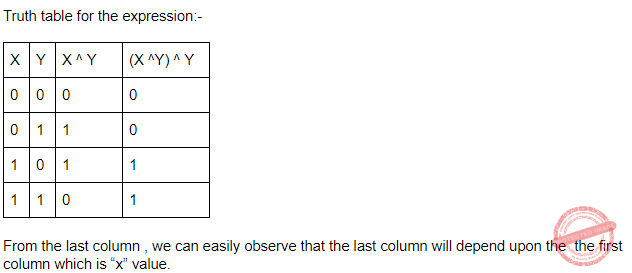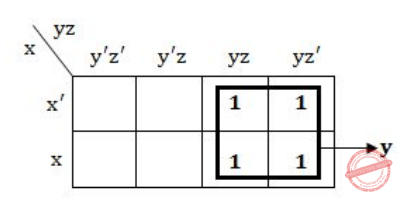## Boolean-Expression

 Question 1

Which one of the following is NOT a valid identity?

 A (x + y) ⊕ z = x ⊕ (y + z) B (x ⊕ y) ⊕ z = x ⊕ (y ⊕ z) C x ⊕ y = x + y, if xy = 0 D x ⊕ y = (xy + x'y')'
Digital-Logic-Design       Boolean-Expression       GATE 2019       Video-Explanation
Question 1 Explanation:
Let x=1, y=1, z=0.
(x+y) ⊕ z = (1+1)⊕ 0 = 1 ⊕ 0 = 1
x ⊕ (y+z) = 1⊕(1+0) = 1 ⊕ 1 = 0
So,
(x+y) ⊕ z ≠ x ⊕ (y+z)
 Question 2
The simplified SOP (Sum of Product) form the Boolean expression (P + Q’ + R’)(P + Q’ + R)(P + Q + R’)
 A (P’Q + R) B (P + Q’R’) C (P Q’ + R ) D (PQ + R)
Digital-Logic-Design       Boolean-Expression       ISRO-2016       Video-Explanation
Question 2 Explanation:Question 3
Evaluate (X XOR Y) XOR Y?
 A All 1’s B All 0’s C X D Y
Digital-Logic-Design       Boolean-Expression       ISRO CS 2011       Video-Explanation
Question 3 Explanation:Question 4
The complement of the Boolean expression AB ( B’C + AC ) is
 A ( A’ + B’ ) + ( B + C’ )( A’ + C’ ) B ( A’ + B’ ) + ( BC’ + A’C’ ) C ( A’ + B’ )( B + C’) + ( A + C’ ) D ( A + B )( B’ + C )( A + C )
Digital-Logic-Design       Boolean-Expression       ISRO CS 2015       Video-Explanation
Question 4 Explanation:
→ Finding complement boolean expression, we require Demorgan’s law.
→ DeMorgan’s law and after complementing:
⇒ ( AB ( B’C + AC ))’
⇒ (A + B)’ + (B’C + AC)’
⇒ (A’ + B’) + ( B’C)’ (AC)’
⇒ (A’ + B’) + ( B + C’)(A’ + C’)
 Question 5
The boolean expression AB + AB’+ A’C + AC is independent of the boolean variable
 A A B B C C D None of these
Digital-Logic-Design       Boolean-Expression       ISRO CS 2015       Video-Explanation
Question 5 Explanation:→ AB + AB' + A'C + AC
= A(B + B') + C(A + A')
= A+C
As the expression is independent of 'B'
 Question 6
The simplified form of the boolean expression: (AB’(C+BD)+A’B’)C is:
 A B’C B A’B’C C AB’C D A’BC
Digital-Logic-Design       Boolean-Expression       KVS 22-12-2018 Part-B
Question 6 Explanation:
(AB’(C+BD)+A’B’)C
(AB'C + AB'BD + A'B')C
(AB'C + A'B')C
AB'C + A'B'C
(A+A') B'C
B'C
 Question 7
Consider the following sequence of instructions :
a=a⊕b, b=a⊕b, a=b⊕a
This Sequence
 A retains the value of the a and b B complements the value of a and b C swap a and b D negates values of a and b
Digital-Logic-Design       Boolean-Expression       UGC NET CS 2005 Dec-Paper-2
Question 7 Explanation:
⇒ a=a⊕b
⇒ b=a ⊕b
= a⊕b ⊕b (Substitute (1))
= 0 ⊕ b
= b
⇒ a = b⊕a
= b⊕a⊕b (Substitute (1))
= a⊕b⊕b
= a⊕0
= a
 Question 8
The logic expression x’yz’+x’yz+xyz’+xyz reduces to :
 A x’z B xyz C y D yz
Digital-Logic-Design       Boolean-Expression       UGC NET CS 2005 june-paper-2
Question 8 Explanation:
We can use two methods to solve this problem.
Method-1 using K-Maps:Method-2 using boolean simplification:
x’yz’+x’yz+xyz’+xyz
= x’y(z’+z)+xy(z’+z)
= x’y + xy
= y(x’+x)
= y
There are 8 questions to complete.

Register Now